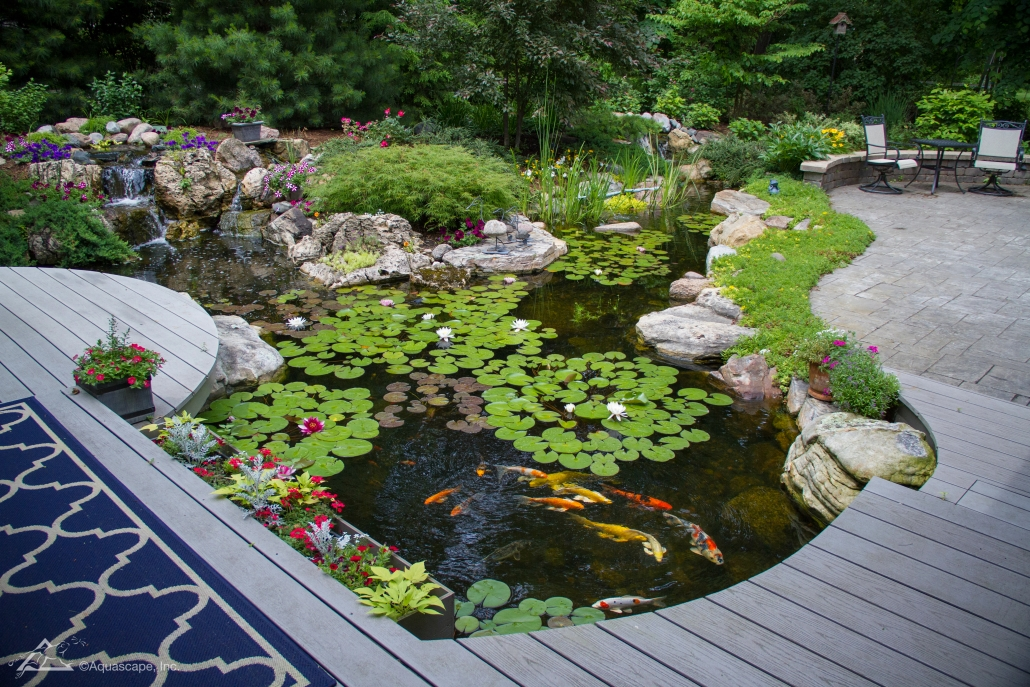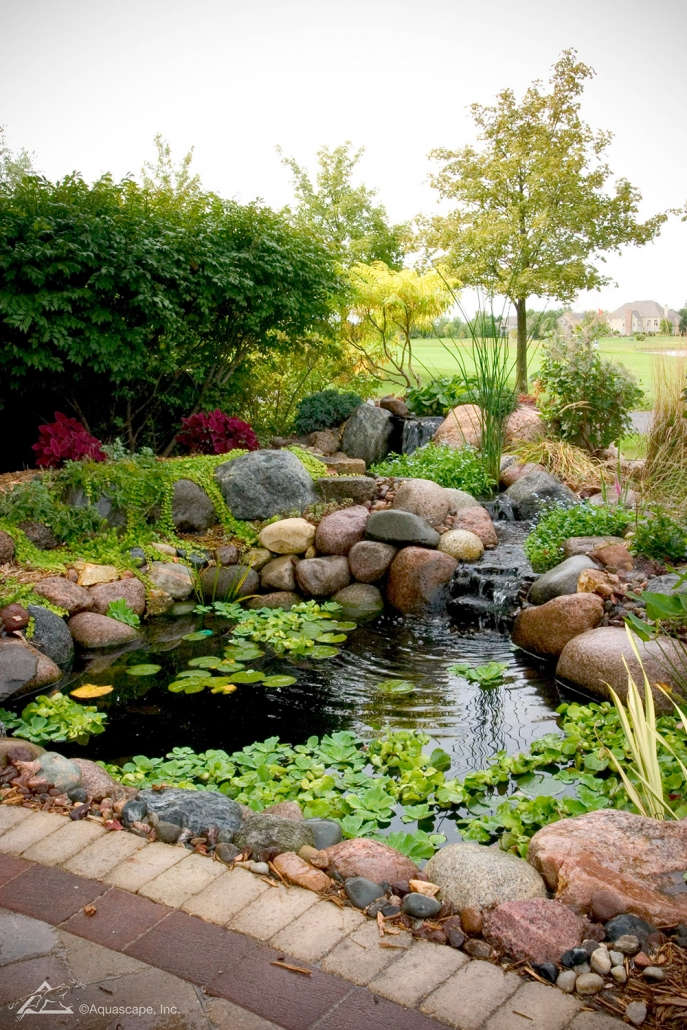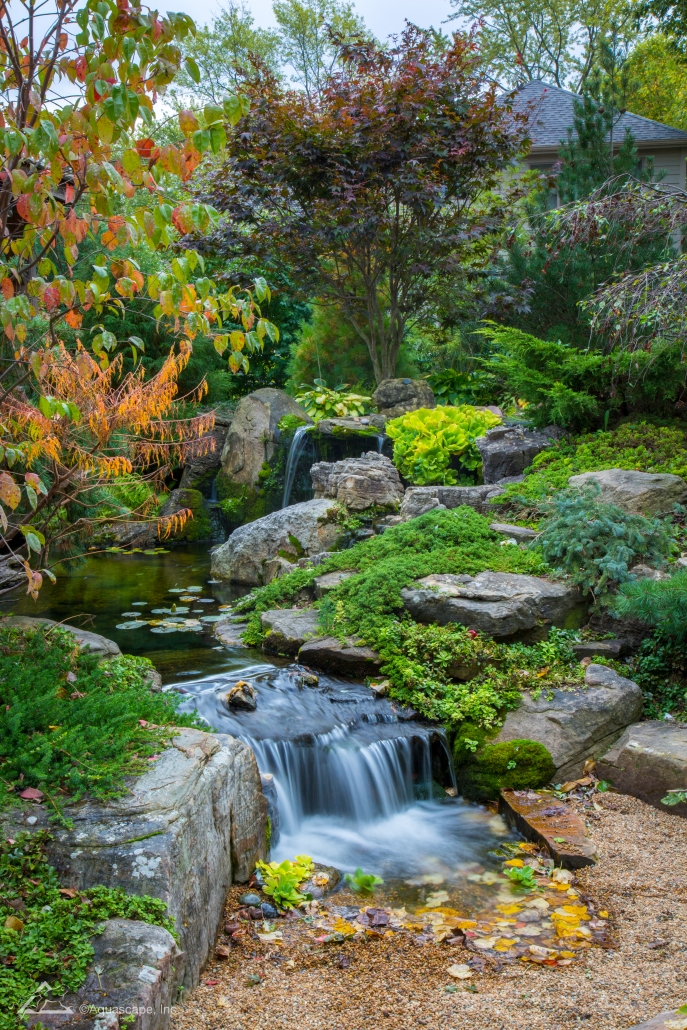### How Long Does a Pond Pump Need to Be on Each Day?

A pond pump and a filter are necessary to maintain pond health. A well-maintained pond adds value to a home, and even though a large pond with fish requires more maintenance than a small pond, it is worth the extra effort, providing movement, color and a dramatic landscape element. A pond’s pump should run 24 hours each day to ensure the pond’s water circulates through the filter to keep the water clear.## Pump Function

A pond pump may be submerged in the pond’s water or sit outside the pond, depending on the pump type, but both types work in the same way. The pump uses spinning blades, known as impellers, to pull pond water through a screenlike intake and expel the water through an outlet pipe. Generally, a large pond has an external pump and a smaller pond or water garden has a submersible pump. Any kind of pump requires regular maintenance of its intake filter and screen to prevent clogging.## Pump Size

Before buying a pond pump, calculate your pond’s water capacity to determine the size of pump required to circulate all of the pond’s water through the pump every one to two hours. Formulas can help you determine your pond’s water capacity in gallons from pond measurements taken in feet. The formula for a rectangular pond is length x width x depth x 7.48 = gallons. For a circular pond, the formula is radius x radius x 3.14 x average depth x 7.48 = gallons. The formula for an irregularly shaped pond requires dividing the pond into easier-to-calculate shapes, figuring the water capacity in gallons for each of those shapes and then multiplying the total sum of those gallons by 231 instead of 7.48.## Operating Cost

A pond that has a pump or other electric equipment requires electricity, and so it will increase your monthly electric cost. Some pumps cost less to operate than other pumps. Reduce the cost of pump operation by using a pump that moves the necessary amount of pond water while using the least amount watts; a watt is a measurement of electrical usage. Calculate the cost of operating a pump by using this formula: Divide the watts by 1,000, and then multiply that figure by 24 hours, the number of days per operation and electricity cost. An example is: 300 watts/1000 x 24 hours x 30 days x 0.08 for electricity cost = \$17.28 as the cost to run the pump for 30 days.## Other Considerations

Mosquitoes can be a problem when a pond is in the landscape, but mosquitoes lay eggs in only stagnant water. A pump can keep the pond water moving, deterring mosquitoes from laying eggs in the water. Even a small amount of moving water can prevent mosquitoes from laying eggs. So if a pump is not feasible, add a fountain bubbler to keep water moving.# How Many Watts Would a 2.5 HP Pump Use?

A pump helps circulate and filter the water in a pool. A pump’s horsepower determines how quickly the pump can process all the water. The goal is for the pump to sufficiently filter all the water in an 8 to 10 hour period, according to Aquascapes. Depending on the size and volume of your pool, you will need more or less horsepower to filter the water in that ideal time frame. Horsepower can be converted to watts, giving you a guesstimate of what your energy costs might be to run your pump.

## Math Conversions

One horsepower is equal to 745.7 watts. When you multiply 745.7 watts by 2.5 hp, you get 1,864.25 watts. Energy costs are calculated in kilowatt-hours, and to get kilowatt-hours, you multiply watts by hours and divide by 1,000. So, if you run your pool pump for 8 hours in one day, 1,864.25 multiplied by 8 and divided by 1,000 equals 14.9 kilowatt-hours. If you run your pump every day for an average of 30 days per month — 30 multiplied by 14.9 — your pump uses 447 kilowatt-hours in a month.

5 + 2 = ?